# Calculate the pH when 9.06 mL of 0.100M NaOH has been added to the original 30.0mL of 0.100M Acetic Acid. Give the answer to two decimal places

Question
1 views

Calculate the pH when 9.06 mL of 0.100M NaOH has been added to the original 30.0mL of 0.100M Acetic Acid. Give the answer to two decimal places

check_circle

Step 1

From the molar equation-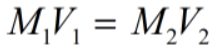M1: molarity of NaOH solution.

V1: volume of NaOH solution.

M2: molarity of acetic acid solution.

V2: volume of acetic acid solution.

Step 2

Moles of sodium hydroxide (n1) can be calculated as-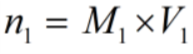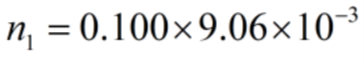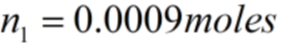Now, moles of acetic acid (n2) can be calculated as-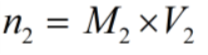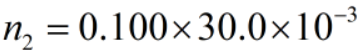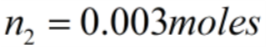Step 3

Since, moles of acetic acid having excess of H+ ions than the moles of sodium hydroxide  having less OH- ions.

Therefore,

Moles (n) of excess of H+ ion will be -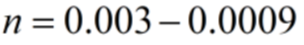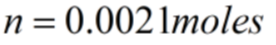Now, calculate concentration of excess of H+ ions by substituting values of excess of H+ ions moles into the molarity equation to obtained as-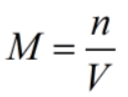V: total volume used during titration that is sum of both volumes.

n: moles of excess H+ ions.

M: molar concentration of excess of H+ ions.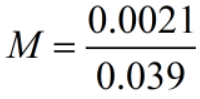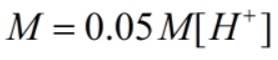...

### Want to see the full answer?

See Solution

#### Want to see this answer and more?

Solutions are written by subject experts who are available 24/7. Questions are typically answered within 1 hour.*

See Solution
*Response times may vary by subject and question.
Tagged in